# varycoef: An R Package to Model Spatially Varying Coefficients

## Introduction

With the R package varycoef we enable the user to analyze spatial data and in a simple, yet versatile way. The underlying idea are spatially varying coefficients (SVC) that extend the linear model

$y_i = x_i^{(1)} \beta_1 + ... + x_i^{(p)} \beta_p + \varepsilon_i$ by allowing the coefficients $$\beta_j, j = 1, ..., p$$ to vary over space. That is, for a location $$s$$ we assume the following model:

$y_i = x_i^{(1)} \beta_1(s) + ... + x_i^{(p)} \beta_p(s) + \varepsilon_i$

In particular, we use so-called Gaussian processes (GP) to define the spatial structure of the coefficients. Therefore, our models are called GP-based SVC models.

In this article, we will show what SVC models are and how to define them. Afterwards, we give a short and illustrative example with synthetic data and show how to apply the methods provided in varycoef. Finally, using the well known data set meuse from the package sp.

### Disclaimer

The analyses and results in this article are meant to introduce the package varycoef and not to be a rigorous statistical analysis of a data set. As this article should make the usage of varycoef as simple as possible, we skip over some of the technical or mathematical details and in some cases abuse notation. For a rigorous definition, please refer to the resources below. Further, the model estimation is performed on rather small data sets to ease computation ($$n < 200$$). We recommend to apply SVC models, particularly with many coefficients, on larger data sets.

### Further References

Our package evolved over time and we present some highlights:

• In Dambon et al. (2021a) we introduce the GP-based SVC model and the methodology on how to estimate them. Further, we provide a comparison on synthetic and real world data with other SVC methodologies.

• In Dambon et al. (2022a) we present an in-depth analysis of Swiss real estate data using GP-based SVC models.

• In Dambon et al. (2022b) we introduce a variable selection method. This is not covered by this article.

• For more information on Gaussian processes and their application on spatial data, please refer to chapter 9 of the “STA330: Modeling Dependent Data” lecture notes by Reinhard Furrer.

### Preliminaries

Before we start, we want to give some prerequisites that you should know about in order to follow the analysis below. Beside a classical linear regression model, we require the knowledge of:

• spatial data and geostatistics
• Gaussian processes and Gaussian random fields
• covariance functions and how the range and variance parameter influence them
• maximum likelihood estimation

### Set up

The varycoef package is available via CRAN or Github. Latter one hosts the most recent version that is released to CRAN on a regular base.

# install from CRAN
install.packages("varycoef")

# install from Github (make sure that you installed the package "devtools")
devtools::install_github("jakobdambon/varycoef")

### Where to find help?

Within the R package, you can use these resources:

# attach package
library(varycoef)

# general package help file
help("varycoef")

# where you find this vignette
vignette("Introduction", package = "varycoef")

You can find this article on the Github repository, too. We continue to add more material and examples.

## Synthetic Data Example

Let’s dive into the analysis of some synthetic data. To ease the visualization, we will work with one-dimensional spatial data, i.e., any location $$s$$ is from the real line $$\mathbb R$$. We want to highlight that are package is not restricted to such analysis and that we can analyze spatial data from higher dimensions, i.e., $$s \in \mathbb R^d$$, where $$d \geq 1$$.

### Model and Data

As mentioned before, an SVC model extends the linear model by allowing the coefficients to vary over space. Therefore, we can write:

$y_i = x_i^{(1)} \beta_1(s) + ... + x_i^{(p)} \beta_p(s) + \varepsilon_i,$ for some location $$s$$ where $$\beta_j(s)$$ indicates the dependence of the $$j$$th coefficient on the space. The coefficients are defined by Gaussian processes, but we skip over this part for now and take a look at a data set that was sampled using the model above. In varycoef, a data set named SVCdata is provided:

str(SVCdata)
## List of 6
##  $y : num [1:500] -1.488 3.901 0.197 -3.326 2.722 ... ##$ X        : num [1:500, 1:2] 1 1 1 1 1 1 1 1 1 1 ...
##  $beta : num [1:500, 1:2] 0.469 0.468 0.452 0.447 0.445 ... ##$ eps      : num [1:500] 0.526 0.311 0.217 0.193 0.646 ...
##  $locs : num [1:500] 0.00465 0.00625 0.06301 0.08216 0.0943 ... ##$ true_pars:'data.frame':   2 obs. of  4 variables:
##   ..$nu : num [1:2] 1.5 1.5 ## ..$ var  : num [1:2] 2 1
##   ..$scale: num [1:2] 3 1 ## ..$ mean : num [1:2] 1 2
help(SVCdata)
# number of observations, number of coefficients
n <- nrow(SVCdata$X); p <- ncol(SVCdata$X)

It consists of the response y, the model matrix X, the locations locs, and the usually unknown true coefficients beta, error eps, and true parameters true_pars. The model matrix is of dimension $$500 \times 2$$, i.e., we have $$500$$ observations and $$p = 2$$ coefficients. The first column of X is identical to 1 to model the intercept.

We will use 100 observations of the data to train the model and leave the remaining 400 out as a test sample, i.e.:

# create data frame
df <- with(SVCdata, data.frame(y = y, x = X[, 2], locs = locs))
set.seed(123)
idTrain <- sort(sample(n, n*prop_train))
df_train <- df[idTrain, ]
df_test <- df[-idTrain, ]

### Exploratory Data Analysis

We plot the part of the data that is usually available to us:

par(mfrow = 1:2)
plot(y ~ x, data = df_train, xlab = "x", ylab = "y",
main = "Scatter Plot of Response and Covariate")
plot(y ~ locs, data = df_train, xlab = "s", ylab = "y",
main = "Scatter Plot of Response and Locations")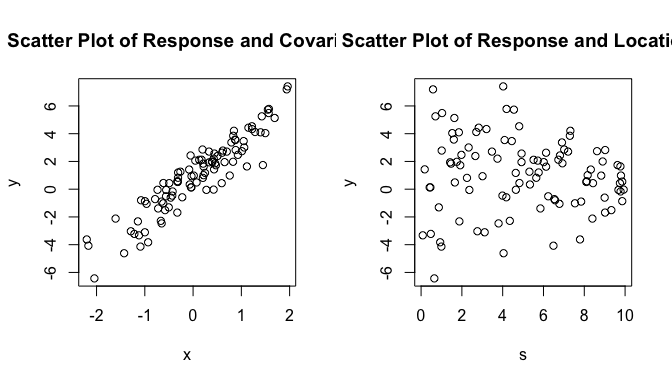par(mfrow = c(1, 1))

We note that there is a clear linear dependency between the covariate and the response. However, there is not a clear spatial structure. We estimate a linear model and analyze the residuals thereof.

fit_lm <- lm(y ~ x, data = df_train)
coef(fit_lm)
## (Intercept)           x
##   0.7703167   2.6885343
# residual plots
par(mfrow = 1:2)
plot(x = fitted(fit_lm), y = resid(fit_lm),
xlab = "Fitted Values", ylab = "Residuals",
main = "Residuals vs Fitted")
abline(h = 0, lty = 2, col = "grey")
plot(x = df_train$locs, y = resid(fit_lm), xlab = "Location", ylab = "Residuals", main = "Residuals vs Locations") abline(h = 0, lty = 2, col = "grey")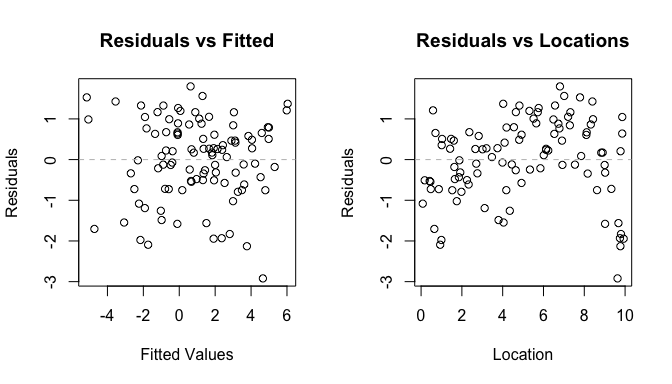par(mfrow = c(1, 1)) Discarding the spatial information, we observe no structure within the residuals (Figure above, LHS). However, if we plot the residuals against there location, we clearly see some spatial structure. Therefore, we will apply the SVC model next. ### SVC Model To estimate an SVC model, we can use the SVC_mle() function almost like the lm() function from above. As the name suggests, we use a maximum likelihood estimation (MLE) for estimating the parameters. For now, we only focus on the mean coefficients, which we obtain by the coef() method. fit_svc <- SVC_mle(y ~ x, data = df_train, locs = df_train$locs)
coef(fit_svc)
## (Intercept)           x
##   0.6490864   2.5468056

The only additional argument that we have to provide explicitly when calling SVC_mle() are the coordinates, i.e., the observation locations. The output is an object of class SVC_mle. We can apply most of the methods that exist for lm objects to out output, too. For instance methods of summary(), fitted(), or resid(). We give the summary() output but do not go into details for now. Further, use the fitted() and residuals() methods to analyze the SVC model. Contrary to a fitted() method for an lm object, the output does not only contain the fitted response, but is a data.frame that contains the spatial deviations from the mean named SVC_1, SVC_2, and so on (see section Model Interpretation below), the response y.pred, and the respective locations loc_1, loc_2, etc. In our case, there is only one column since the locations are from a one-dimensional domain.

# summary output
summary(fit_svc)
##
## Call:
## SVC_mle.formula(formula = y ~ x, data = df_train, locs = df_train$locs) ## ## Fitting a GP-based SVC model with 2 fixed effect(s) and 2 SVC(s) ## using 100 observations at 100 different locations / coordinates. ## ## Residuals: ## Min. 1st Qu. Median 3rd Qu. Max. ## -1.25497 -0.26972 0.01098 0.30372 0.98823 ## ## Residual standard error: 0.4307 ## Multiple R-squared: 0.9736, BIC: 231.8 ## ## ## Coefficients of fixed effect(s): ## Estimate Std. Error Z value Pr(>|Z|) ## (Intercept) 0.6491 0.2838 2.287 0.0222 * ## x 2.5468 0.3499 7.280 3.35e-13 *** ## --- ## Signif. codes: 0 '***' 0.001 '**' 0.01 '*' 0.05 '.' 0.1 ' ' 1 ## ## ## Covariance parameters of the SVC(s): ## Estimate Std. Error W value Pr(>W) ## (Intercept).range 1.68358 1.44902 NA NA ## (Intercept).var 0.25786 0.15979 2.604 0.1066 ## x.range 1.31196 1.16981 NA NA ## x.var 0.51061 0.31030 2.708 0.0999 . ## nugget.var 0.26822 0.05479 NA NA ## --- ## Signif. codes: 0 '***' 0.001 '**' 0.01 '*' 0.05 '.' 0.1 ' ' 1 ## ## The covariance parameters were estimated using ## exponential covariance functions. ## No covariance tapering applied. ## ## ## MLE: ## The MLE terminated after 76 function evaluations with convergence code 0 ## (0 meaning that the optimization was succesful). ## The final log likelihood value is -106.7. # fitted output head(fitted(fit_svc)) ## SVC_1 SVC_2 y.pred loc_1 ## 4 -0.3353062 0.5712240 -3.1805285 0.0821552 ## 7 -0.3390177 0.5691786 1.6638651 0.1776581 ## 13 -0.2881019 0.6498610 0.2224001 0.4205953 ## 14 -0.2775242 0.6628253 0.2605299 0.4555650 ## 16 -0.2681113 0.6705916 -3.5324973 0.4766363 ## 23 -0.2584885 0.8309101 6.9425422 0.5847849 # residual plots par(mfrow = 1:2) plot(x = fitted(fit_svc)$y.pred, y = resid(fit_svc),
xlab = "Fitted Values", ylab = "Residuals",
main = "Residuals vs Fitted")
abline(h = 0, lty = 2, col = "grey")
plot(x = df_train$locs, y = resid(fit_svc), xlab = "Location", ylab = "Residuals", main = "Residuals vs Locations") abline(h = 0, lty = 2, col = "grey")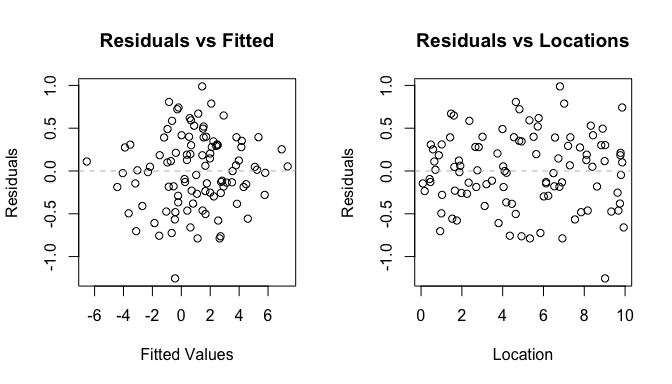par(mfrow = c(1, 1)) Compared to the output and residuals of the linear models, we immediately see that the residuals of the SVC model are smaller in range and do not have a spatial structure. They are both with respect to fitted values and locations distributed around zero and homoscedastic. ### Comparison of Models We end this synthetic data example by comparing the linear with the SVC model. We already saw some advantages of the SVC model when investigating the residuals. Now, we take a look at the quality of the model fit, the model interpretation, and the predictive performance. #### Model Fit We can compare the models by the log likelihood, Akaike’s or the Bayesian information criterion (AIC and BIC, respectively). Again, the corresponding methods are available: kable(data.frame( Model = c("linear", "SVC"), # using method logLik log Likelihood = round(as.numeric(c(logLik(fit_lm), logLik(fit_svc))), 2), # using method AIC AIC = round(c(AIC(fit_lm), AIC(fit_svc)), 2), # using method BIC BIC = round(c(BIC(fit_lm), BIC(fit_svc)), 2) )) Model log.Likelihood AIC BIC linear -139.92 285.85 293.66 SVC -106.70 284.65 231.82 In all four metrics the SVC model outperforms the linear model, i.e., the log likelihood is larger and the two information criteria are smaller. #### Visualization of Coefficients While the linear model estimates constant coefficients $$\beta_j$$, contrary and as the name suggests, the SVC model’s coefficients vary over space $$\beta_j(s)$$. That is why the fitted() method does not only return the fitted response, but also the fitted coefficients and at their given locations: head(fitted(fit_svc)) ## SVC_1 SVC_2 y.pred loc_1 ## 4 -0.3353062 0.5712240 -3.1805285 0.0821552 ## 7 -0.3390177 0.5691786 1.6638651 0.1776581 ## 13 -0.2881019 0.6498610 0.2224001 0.4205953 ## 14 -0.2775242 0.6628253 0.2605299 0.4555650 ## 16 -0.2681113 0.6705916 -3.5324973 0.4766363 ## 23 -0.2584885 0.8309101 6.9425422 0.5847849 Therefore, the SVC mentioned above is the sum of the mean value from the method coef() which we name $$\mu_j$$ and the zero-mean spatial deviations from above which we name $$\eta_j(s)$$, i.e., $$\beta_j(s) = \mu_j + \eta_j(s)$$. We visualize the coefficients at their respective locations: mat_coef <- cbind( # constant coefficients from lm lin1 = coef(fit_lm), lin2 = coef(fit_lm), # SVCs svc1 = coef(fit_svc) + fitted(fit_svc)[, 1], svc2 = coef(fit_svc) + fitted(fit_svc)[, 2] ) matplot( x = df_train$locs,
y = mat_coef, pch = c(1, 2, 1, 2), col = c(1, 1, 2, 2),
xlab = "Location", ylab = "Beta", main = "Estimated Coefficients")
legend("topright", legend = c("Intercept", "covariate x", "linear model", "SVC model"),
pch = c(1, 2, 19, 19), col = c("grey", "grey", "black", "red"))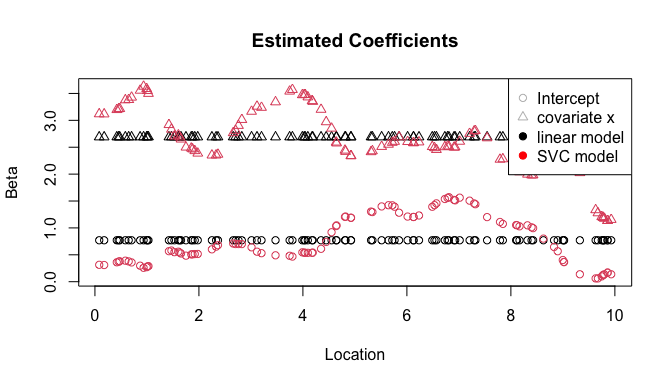#### Spatial Prediction

We use the entire data set to compute the in- and out-of-sample rooted mean square error (RMSE) for the response for both the linear and SVC model. Here we rely on the predict() methods. Further, we can compare the predicted coefficients with the true coefficients provided in SVCdata, something that we usually cannot do.

# using method predict with whole data and corresponding locations
df_svc_pred <- predict(fit_svc, newdata = df, newlocs = df$locs) # combining mean values and deviations mat_coef_pred <- cbind( svc1_pred = coef(fit_svc) + df_svc_pred[, 1], svc2_pred = coef(fit_svc) + df_svc_pred[, 2] ) # plot matplot(x = df$locs, y = mat_coef_pred,
xlab = "Location", ylab = "Beta",
main = "Predicted vs Actual Coefficients",
col = c(1, 2), lty = c(1, 1), type = "l")
points(x = df$locs, y = SVCdata$beta[, 1], col = 1, pch = ".")
points(x = df$locs, y = SVCdata$beta[, 2], col = 2, pch = ".")
legend("topright", legend = c("Intercept", "Covariate", "Actual"),
col = c("black", "red", "black"),
pch = c(NA, NA, "."),
lty = c(1, 1, NA))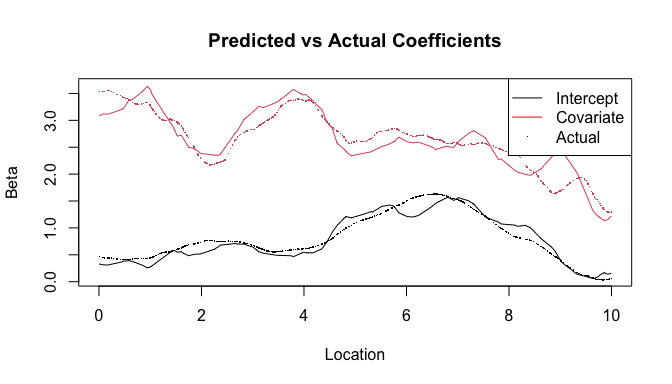#### Model Interpretation

The linear model is constant and the same for each location, i.e.:

$y = \beta_1 + \beta_2\cdot x + \varepsilon = 0.77 + 2.69 \cdot x + \varepsilon$ Here, the SVC model can be interpreted in a similar way where on average, it is simply the mean coefficient value with some location specific deviation $$\eta_j(s)$$, i.e.:

$y = \beta_1(s) + \beta_2(s)\cdot x + \varepsilon = \bigl(0.65 + \eta_1(s)\bigr) + \bigl(2.55+ \eta_2(s) \bigr) \cdot x + \varepsilon$

Say we are interested in a particular position, like:

(s_id <- sample(n, 1))
##  159

The coordinate is $$s_{159} = 3.12$$. We can extract the deviations $$\eta_j(s)$$ and simply add them to the model. We receive the following location specific model.

$y = \beta_1(s_{159}) + \beta_2(s_{159})\cdot x + \varepsilon = \bigl(0.65 -0.09\bigr) + \bigl(2.55+ 0.71\bigr) \cdot x + \varepsilon = 0.56 + 3.26 \cdot x + \varepsilon$ The remaining comparison of the two model at the given location is as usual. Between the both models we see that the intercept of the linear model is larger and the coefficient of $$x$$ is smaller than the SVC model’s intercept and coefficient of $$x$$, respectively.

#### Predictive Performance

Finally, we compare the prediction errors of the model. We compute the rooted mean squared errors (RMSE) for both models and both the training and testing data.

SE_lm <- (predict(fit_lm, newdata = df) - df$y)^2 # df_svc_pred from above SE_svc <- (df_svc_pred$y.pred - df$y)^2 kable(data.frame( model = c("linear", "SVC"), in-sample RMSE = round(sqrt(c(mean(SE_lm[idTrain]), mean(SE_svc[idTrain]))), 3), out-of-sample RMSE = round(sqrt(c(mean(SE_lm[-idTrain]), mean(SE_svc[-idTrain]))), 3) )) model in.sample.RMSE out.of.sample.RMSE linear 0.980 0.791 SVC 0.429 0.558 We notice a significant difference in both RMSE between the models. On the training data, i.e., the in-sample RMSE, we observe and improvement by more than 50%. This is quite common since the SVC model has a higher flexibility. Therefore, it is very pleasing to see that even on the testing data, i.e., the out-of-sample RMSE, the SVC model still improves the RMSE by almost 30% compared to the linear model. ## Meuse Data Set Example We now turn to a real world data set, the meuse data from the package sp. library(sp) # attach sp and load data data("meuse") # documentation help("meuse") # overview summary(meuse) ## x y cadmium copper ## Min. :178605 Min. :329714 Min. : 0.200 Min. : 14.00 ## 1st Qu.:179371 1st Qu.:330762 1st Qu.: 0.800 1st Qu.: 23.00 ## Median :179991 Median :331633 Median : 2.100 Median : 31.00 ## Mean :180005 Mean :331635 Mean : 3.246 Mean : 40.32 ## 3rd Qu.:180630 3rd Qu.:332463 3rd Qu.: 3.850 3rd Qu.: 49.50 ## Max. :181390 Max. :333611 Max. :18.100 Max. :128.00 ## ## lead zinc elev dist ## Min. : 37.0 Min. : 113.0 Min. : 5.180 Min. :0.00000 ## 1st Qu.: 72.5 1st Qu.: 198.0 1st Qu.: 7.546 1st Qu.:0.07569 ## Median :123.0 Median : 326.0 Median : 8.180 Median :0.21184 ## Mean :153.4 Mean : 469.7 Mean : 8.165 Mean :0.24002 ## 3rd Qu.:207.0 3rd Qu.: 674.5 3rd Qu.: 8.955 3rd Qu.:0.36407 ## Max. :654.0 Max. :1839.0 Max. :10.520 Max. :0.88039 ## ## om ffreq soil lime landuse dist.m ## Min. : 1.000 1:84 1:97 0:111 W :50 Min. : 10.0 ## 1st Qu.: 5.300 2:48 2:46 1: 44 Ah :39 1st Qu.: 80.0 ## Median : 6.900 3:23 3:12 Am :22 Median : 270.0 ## Mean : 7.478 Fw :10 Mean : 290.3 ## 3rd Qu.: 9.000 Ab : 8 3rd Qu.: 450.0 ## Max. :17.000 (Other):25 Max. :1000.0 ## NA's :2 NA's : 1 dim(meuse) ##  155 14 Our goal is to model the log cadmium measurements using the following independent variables: • dist, i.e. the normalized distance to the river Meuse. • lime, which is a 2-level factor indicating the presence of lime. • elev, i.e. the relative elevation above the local river bed. This provides us a model with “cheap” covariates and we can regress our variable of interest on them. df_meuse <- meuse[, c("dist", "lime", "elev")] df_meuse$l_cad <- log(meuse$cadmium) df_meuse$lime <- as.numeric(as.character(df_meuse\$lime))
locs <- as.matrix(meuse[, c("x", "y")])

### Exploratory Data Analysis

First, we plot the log Cadmium measurements at their respective locations. The color ranges from yellow (high Cadmium measurements) to black (low Cadmium measurements).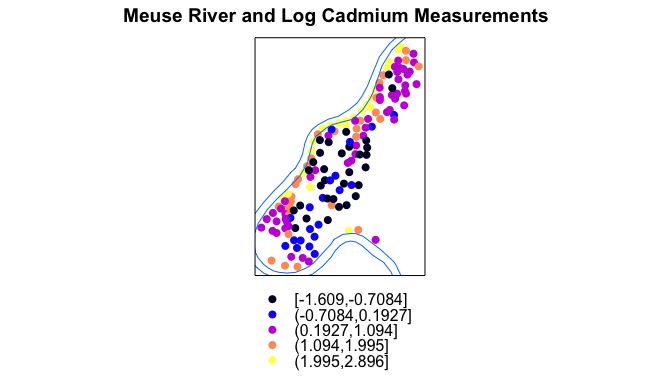Generally, the values for l_cad are highest close to the river Meuse. However, there is some spatial structure comparing the center of all observations to the Northern part. Therefore, we expect the dist covariate to be an important regressor. Omitting the spatial structure, we can also look at a pairs plot.

pairs(df_meuse)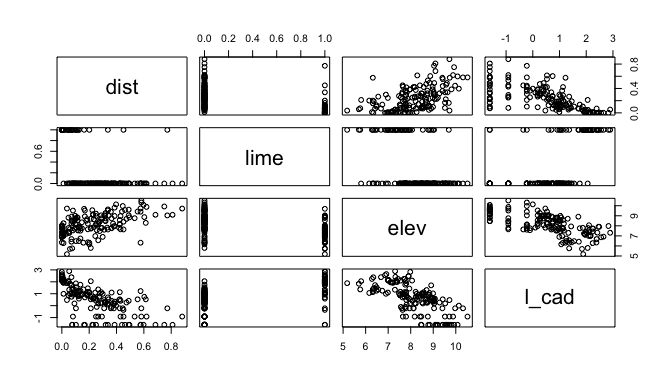Indeed, we note linear relationships between l_cad on the one hand and elev as well as dist on the other hand. Further, when lime is equal to 1, i.e., there is lime present in the soil, the Cadmium measurements are higher.

### Linear Model

fit_lm <- lm(l_cad ~ ., data = df_meuse)
coef(fit_lm)
## (Intercept)        dist        lime        elev
##   4.7508453  -2.0449732   0.5763247  -0.4730394

The residual analysis shows:

oldpar <- par(mfrow = c(1, 2))
plot(fit_lm, which = 1:2)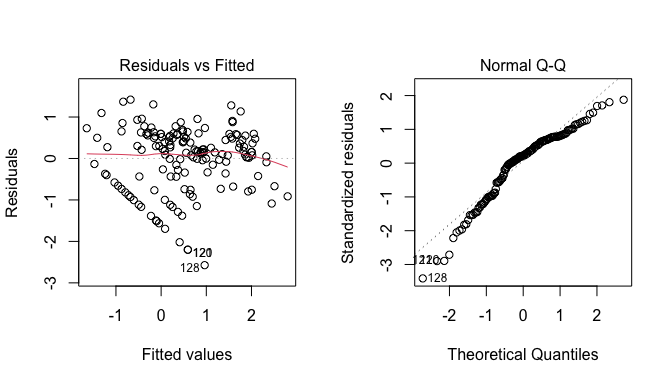par(oldpar)

The spatial distribution of the residuals is the following: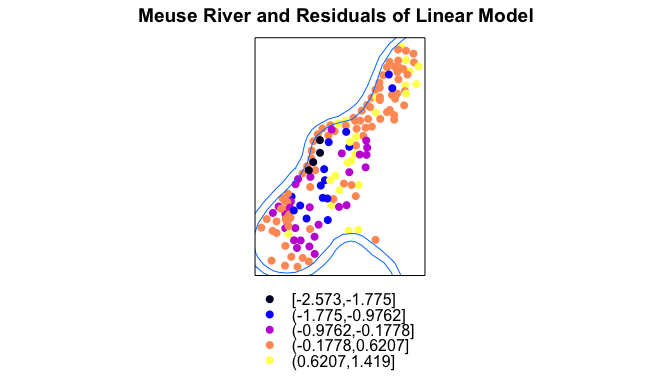One can observe that there is a spatial structure in the residuals. This motivates us to use an SVC model.

### SVC Model

The call to estimate the SVC model is again quite similar to the lm() call from above. However, we specify further arguments for the MLE using the control argument. First, we are using an optimization over the profiled likelihood (profileLik = TRUE) and second, we apply parameter scaling (parscale = TRUE) in the numeric optimization. Please check the corresponding help file help("SVC_mle_control") for more details.

fit_svc <- SVC_mle(l_cad ~ ., data = df_meuse, locs = locs,
control = SVC_mle_control(
profileLik = TRUE,
parscale = TRUE
))
coef(fit_svc)
## (Intercept)        dist        lime        elev
##   5.6523164  -2.3143392   0.5731665  -0.5711638

The obtained mean coefficient values of the linear and SVC model are quite similar, but this does not come as a surprise.

(Intercept) dist lime elev
linear 4.751 -2.045 0.576 -0.473
SVC 5.652 -2.314 0.573 -0.571

### Predictions

Additionally to the meuse data the sp package also contains another data set called meuse.grid that contains a 40 by 40 meter spaced grid of the entire study area along the Meuse river. We can use the locations to predict the SVCs. However, the covariates elev and lime are missing. Therefore, we cannot predict the response. Again, the fitted SVC values are only the deviations from the mean. We observe that the SVC for elev is in fact constant.

# study area
data("meuse.grid")
# prediction
df_svc_pred <- predict(fit_svc, newlocs = as.matrix(meuse.grid[, c("x", "y")]))
colnames(df_svc_pred)[1:4] <- c("Intercept", "dist", "lime", "elev")
head(df_svc_pred)
##   Intercept       dist       lime elev  loc_1  loc_2
## 1 0.2004922 0.09882029 0.04280647    0 181180 333740
## 2 0.2169662 0.11452429 0.04797774    0 181140 333700
## 3 0.2132619 0.11474298 0.04543971    0 181180 333700
## 4 0.2081517 0.11375315 0.04257064    0 181220 333700
## 5 0.2340236 0.13166165 0.05360972    0 181100 333660
## 6 0.2310287 0.13362685 0.05089961    0 181140 333660

The attentive reader might have noticed that the elev column only contains 0. Therefore, there are no deviations and the respective coefficient is constant and our method is capable to estimate constant coefficients within the MLE. There exists a selection method to not only select the varying coefficients, but to also select the mean value, i.e., the fixed effect. Please refer to Dambon et al. (2022b) for further information.

## Conclusion

SVC models are a powerful tool to analyze spatial data. With the R package varycoef, we provide an accessible and easy way to apply GP-based SVC models that is very close to the classical lm experience. If you have further questions or issues, please visit our Github repository.